Open in App
Not now

# C Program for Merge Sort

• Difficulty Level : Easy
• Last Updated : 03 Jun, 2022

Like QuickSort, Merge Sort is a Divide and Conquer algorithm. It divides the input array into two halves, calls itself for the two halves, and then it merges the two sorted halves. The merge() function is used for merging two halves. The merge(arr, l, m, r) is a key process that assumes that arr[l..m] and arr[m+1..r] are sorted and merges the two sorted sub-arrays into one.

Pseudocode :

```• Declare left variable to 0 and right variable to n-1
• Find mid by medium formula. mid = (left+right)/2
• Call merge sort on (left,mid)
• Call merge sort on (mid+1,rear)
• Continue till left is less than right
• Then call merge function to perform merge sort.```

Algorithm:

```Step 1: Start
Step 2: Declare an array and left, right, mid variable
Step 3: Perform merge function.
mergesort(array,left,right)
mergesort (array, left, right)
if left > right
return
mid= (left+right)/2
mergesort(array, left, mid)
mergesort(array, mid+1, right)
merge(array, left, mid, right)
Step 4: Stop```

See the following C implementation for details.

MergeSort(arr[], l,  r)

If r > l

• Find the middle point to divide the array into two halves:
• middle m = l + (r – l)/2
• Call mergeSort for first half:
• Call mergeSort(arr, l, m)
• Call mergeSort for second half:
• Call mergeSort(arr, m + 1, r)
• Merge the two halves sorted in step 2 and 3:
• Call merge(arr, l, m, r)

### How Merge sort Works?

To know the functioning of merge sort, lets consider an array arr[] = {38, 27, 43, 3, 9, 82, 10}

• At first, check if the left index of array is less than the right index, if yes then calculate its mid point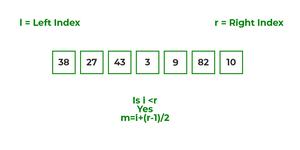• Now, as we already know that merge sort first divides the whole array iteratively into equal halves, unless the atomic values are achieved.
• Here, we see that an array of 7 items is divided into two arrays of size 4 and 3 respectively.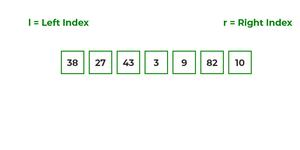• Now, again find that is left index is less than the right index for both arrays, if found yes, then again calculate mid points for both the arrays.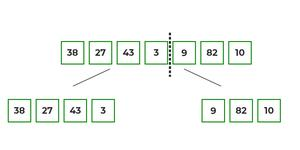• Now, further divide these two arrays into further halves, until the atomic units of the array is reached and further division is not possible.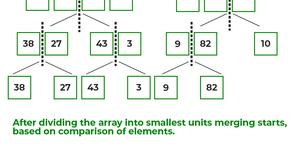• After dividing the array into smallest units, start merging the elements again based on comparison of size of elements
• Firstly, compare the element for each list and then combine them into another list in a sorted manner.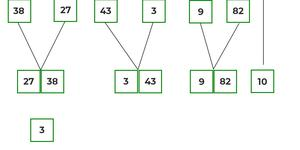• After the final merging, the list looks like this: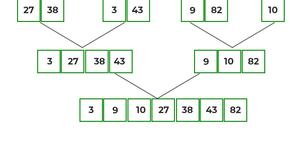Refer to below illustrations for further clarity:

The following diagram shows the complete merge sort process for an example array {38, 27, 43, 3, 9, 82, 10}.

If we take a closer look at the diagram, we can see that the array is recursively divided into two halves till the size becomes

• Once the size becomes 1, the merge processes come into action and start merging arrays back till the complete array is merged.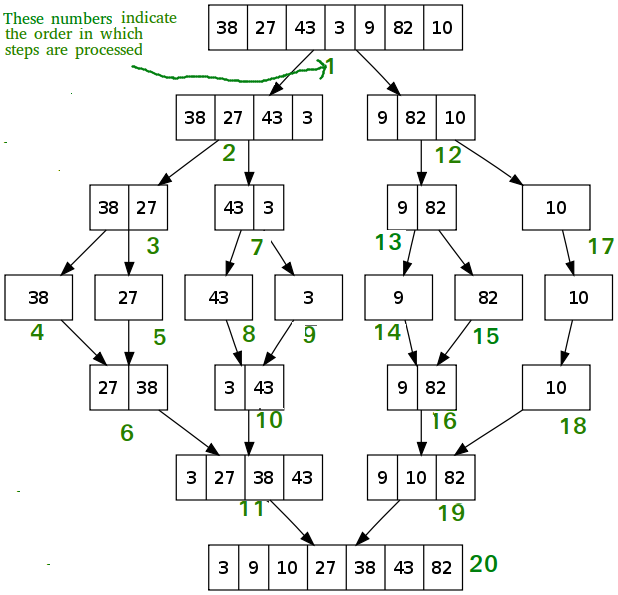## C

 `// C program for Merge Sort ``#include ``#include `` ` `// Merges two subarrays of arr[].``// First subarray is arr[l..m]``// Second subarray is arr[m+1..r]``void` `merge(``int` `arr[], ``int` `l, ``           ``int` `m, ``int` `r)``{``    ``int` `i, j, k;``    ``int` `n1 = m - l + 1;``    ``int` `n2 = r - m;`` ` `    ``// Create temp arrays``    ``int` `L[n1], R[n2];`` ` `    ``// Copy data to temp arrays ``    ``// L[] and R[] ``    ``for` `(i = 0; i < n1; i++)``        ``L[i] = arr[l + i];``    ``for` `(j = 0; j < n2; j++)``        ``R[j] = arr[m + 1 + j];`` ` `    ``// Merge the temp arrays back ``    ``// into arr[l..r]``    ``// Initial index of first subarray``    ``i = 0; `` ` `    ``// Initial index of second subarray``    ``j = 0; `` ` `    ``// Initial index of merged subarray``    ``k = l; ``    ``while` `(i < n1 && j < n2) ``    ``{``        ``if` `(L[i] <= R[j]) ``        ``{``            ``arr[k] = L[i];``            ``i++;``        ``}``        ``else` `        ``{``            ``arr[k] = R[j];``            ``j++;``        ``}``        ``k++;``    ``}`` ` `    ``// Copy the remaining elements ``    ``// of L[], if there are any``    ``while` `(i < n1) {``        ``arr[k] = L[i];``        ``i++;``        ``k++;``    ``}`` ` `    ``// Copy the remaining elements of ``    ``// R[], if there are any ``    ``while` `(j < n2) ``    ``{``        ``arr[k] = R[j];``        ``j++;``        ``k++;``    ``}``}`` ` `// l is for left index and r is ``// right index of the sub-array ``// of arr to be sorted ``void` `mergeSort(``int` `arr[], ``               ``int` `l, ``int` `r)``{``    ``if` `(l < r) ``    ``{``        ``// Same as (l+r)/2, but avoids ``        ``// overflow for large l and h``        ``int` `m = l + (r - l) / 2;`` ` `        ``// Sort first and second halves``        ``mergeSort(arr, l, m);``        ``mergeSort(arr, m + 1, r);`` ` `        ``merge(arr, l, m, r);``    ``}``}`` ` `// UTILITY FUNCTIONS ``// Function to print an array ``void` `printArray(``int` `A[], ``int` `size)``{``    ``int` `i;``    ``for` `(i = 0; i < size; i++)``        ``printf``(``"%d "``, A[i]);``    ``printf``(``"\n"``);``}`` ` `// Driver code``int` `main()``{``    ``int` `arr[] = {12, 11, 13, 5, 6, 7};``    ``int` `arr_size = ``sizeof``(arr) / ``sizeof``(arr);`` ` `    ``printf``(``"Given array is \n"``);``    ``printArray(arr, arr_size);`` ` `    ``mergeSort(arr, 0, arr_size - 1);`` ` `    ``printf``(``"\nSorted array is \n"``);``    ``printArray(arr, arr_size);``    ``return` `0;``}`

Output:

```Given array is
12 11 13 5 6 7
Sorted array is
5 6 7 11 12 13 ```

Time Complexity: O(n logn),  Sorting arrays on different machines. Merge Sort is a recursive algorithm and time complexity can be expressed as following recurrence relation.
T(n) = 2T(n/2) + θ(n)

The above recurrence can be solved either using the Recurrence Tree method or the Master method. It falls in case II of Master Method and the solution of the recurrence is θ(nLogn). Time complexity of Merge Sort is  θ(nLogn) in all 3 cases (worst, average and best) as merge sort always divides the array into two halves and takes linear time to merge two halves.
Auxiliary Space: O(n)

Space Complexity :

• In merge sort all elements are copied into an auxiliary array
• so N auxiliary space is required for merge sort.

### Is Merge sort In Place?

No in a typical implementation

### Is Merge sort Stable:

Yes, merge sort is stabe.

### Applications of Merge Sort:

• Merge Sort is useful for sorting linked lists in O(nLogn) time. In the case of linked lists, the case is different mainly due to the difference in memory allocation of arrays and linked lists. Unlike arrays, linked list nodes may not be adjacent in memory. Unlike an array, in the linked list, we can insert items in the middle in O(1) extra space and O(1) time. Therefore, the merge operation of merge sort can be implemented without extra space for linked lists.
In arrays, we can do random access as elements are contiguous in memory. Let us say we have an integer (4-byte) array A and let the address of A be x then to access A[i], we can directly access the memory at (x + i*4). Unlike arrays, we can not do random access in the linked list. Quick Sort requires a lot of this kind of access. In a linked list to access i’th index, we have to travel each and every node from the head to i’th node as we don’t have a continuous block of memory. Therefore, the overhead increases for quicksort. Merge sort accesses data sequentially and the need of random access is low.
• Inversion Count Problem
• Used in External Sorting

### Drawbacks of Merge Sort:

• Slower comparative to the other sort algorithms for smaller tasks.
• Merge sort algorithm requires an additional memory space of 0(n) for the temporary array.
• It goes through the whole process even if the array is sorted.

Please refer complete article on Merge Sort for more details!

My Personal Notes arrow_drop_up